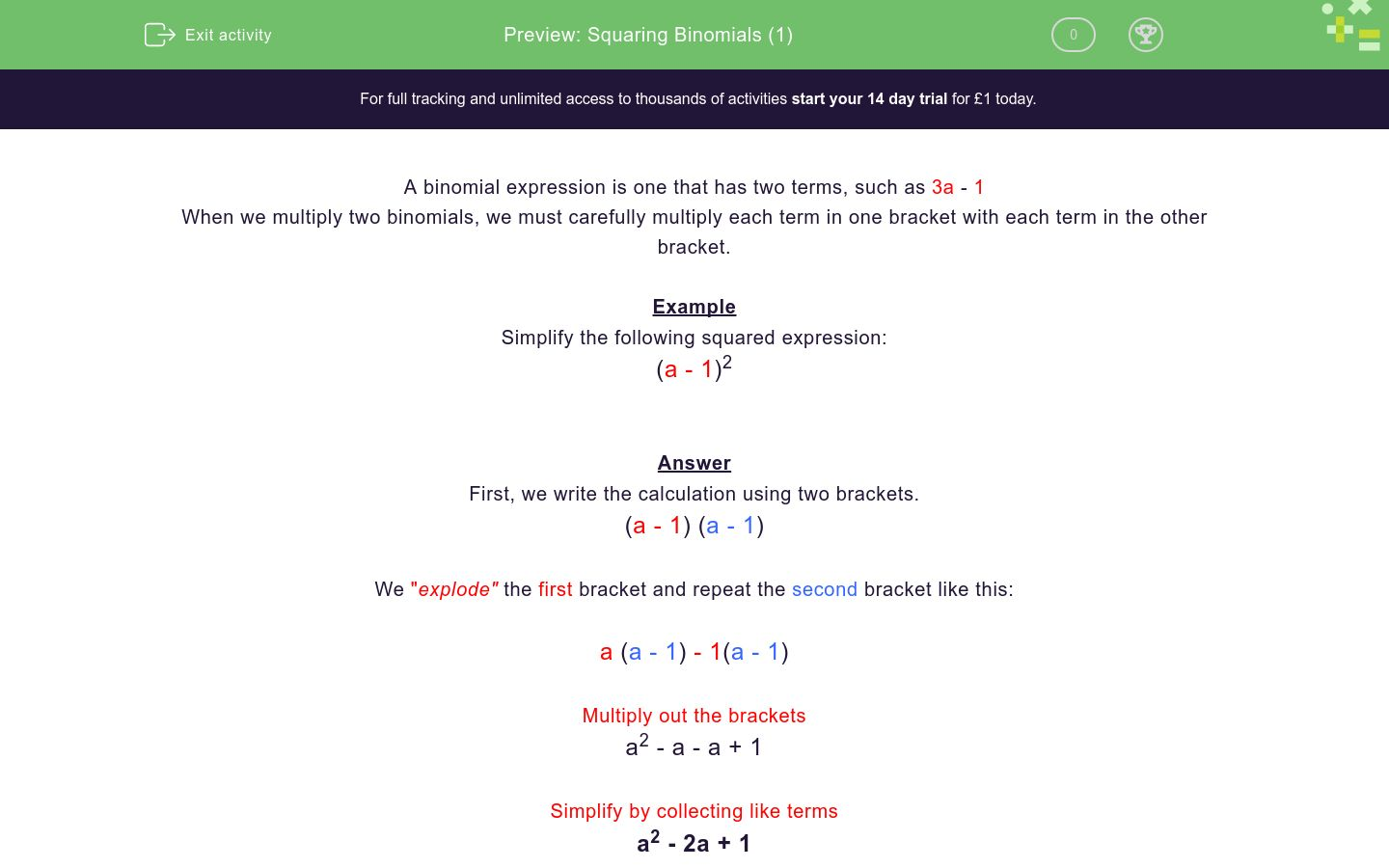# Squaring Binomials (1)

In this worksheet, students square binomial expressions.Key stage:  KS 4

Curriculum topic:  Algebra

Difficulty level:### QUESTION 1 of 10

A binomial expression is one that has two terms, such as 3a - 1

When we multiply two binomials, we must carefully multiply each term in one bracket with each term in the other bracket.

Example

Simplify the following squared expression:

(a - 1)2

First, we write the calculation using two brackets.

(a - 1) (a - 1)

We "explode" the first bracket and repeat the second bracket like this:

a (a - 1) - 1(a - 1)

Multiply out the brackets

a2 - a - a + 1

Simplify by collecting like terms

a2 - 2a + 1

Simplify the following squared expression:

(a + 1)2

a2+2a +1

a2+5a +2

a2+3a +1

a2+2a +2

Simplify the following squared expression:

(a - 2)2

a2+2a +4

2a2+4a +6

a2+4a +2

a2-4a +4

Simplify the following squared expression:

(a - 3)2

a2+9a +6

a2-6a +9

3a2+6a +9

3a2+6a -9

Simplify the following squared expression:

(a + 2)2

a2+4a +4

a2+3a -4

4a2+a -2

a2+4a +2

Simplify the following squared expression:

(a + 3)2

a2+9a +6

3a2+9a -6

3a2+6a +6

a2+6a +9

Simplify the following squared expression:

(a - 5)2

5a2+10a -5

5a2-a -25

4a2+5a -25

a2-10a +25

Simplify the following squared expression:

(a - 10)2

10a2-100a

a2+20a -100

a2-20a +100

a2+10a -20

Simplify the following squared expression:

(a + 8)2

8a2+64

a2-8a -8

a2+16a +64

a2+64

Simplify the following squared expression:

(a - 7)2

a2-14a +49

a2-49

a2+49

a2-14a -49

Simplify the following squared expression:

(a - 11)2

11a2+11a -11

a2-121a +22

a2-11a +121

a2-22a +121

• Question 1

Simplify the following squared expression:

(a + 1)2

a2+2a +1
• Question 2

Simplify the following squared expression:

(a - 2)2

a2-4a +4
• Question 3

Simplify the following squared expression:

(a - 3)2

a2-6a +9
• Question 4

Simplify the following squared expression:

(a + 2)2

a2+4a +4
• Question 5

Simplify the following squared expression:

(a + 3)2

a2+6a +9
• Question 6

Simplify the following squared expression:

(a - 5)2

a2-10a +25
• Question 7

Simplify the following squared expression:

(a - 10)2

a2-20a +100
• Question 8

Simplify the following squared expression:

(a + 8)2

a2+16a +64
• Question 9

Simplify the following squared expression:

(a - 7)2

a2-14a +49
• Question 10

Simplify the following squared expression:

(a - 11)2